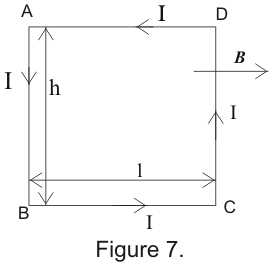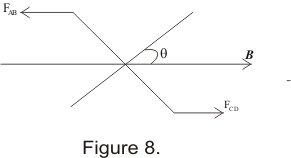# Torque on a current carrying rectangular loop in a magnetic field|Magnetism

## Torque on a current carrying rectangular loop in a magnetic field

• Consider a rectangular loop ABCD being suspended in a uniform magnetic field B and direction of B is parallel to the plane of the coil as shown below in the figure• Magnitude of force on side AM according to the equation(13) is
FAB=IhB ( angle between I and B is 900)
And direction of force as calculated from the right hand palm rule would be normal to the paper in the upwards direction
• Similarly magnitude of force on CD is
FCD=ihB
and direction of FCD is normal to the page but in the downwards direction going into the page
• The forces FAB and FCD are equal in magnitude and opposite in direction and hence they constitute a couple
• Torque τexerted by this couple on rectangular loop is
τ=IhlB
Since torque = one of the force * perpendicular distance between them
• No force acts on the side BC since current element makes an angle θ=0 with B due to which the product (ILXB) becomes equal to zero
• Similarly on the side DA ,no magnetic force acts since current element makes an angle θ=1800 with B
• Thus total torque on rectangular current loop is
τ=IhlB
=IAB                   --(15)
Where A=hl is the area of the loop
• If the coil having N rectangular loop is placed in magnetic field then torque is given by
τ=NIAB                   ----(16)
• Again if the normal to the plane of coil makes an angle θ with the uniform magnetic field as shown below in the figure thenτ=NIABsinθ
• We know that when an electric dipole is placed in external electric field then torque experienced by the dipole is
τ=P X E=PEsinθ
Where P is the electric dipole moment
• comparing expression for torque experienced by electric dipole with the expression for torque on a current loop i.e ,
τ=(NIA)Bsinθ
if we take NIA as magnetic dipole moment (m) analogous to electric dipole moment (p),we have
m=NIA                   -- (18)
then
τ=m X B                   -- (19)
• The coil thus behaves as a magnetic dipole
• The direction of magnetic dipole moment lies along the axis of the loop
• This torque tends to rotate the coil about its own axis .Its value changes with angle between the plane of the coil and the direction of the magnetic field
• Unit of magnetic moment is Ampere.meter2 (Am2)
• Equation (18) and (19) are obtained by considering a rectangular loop but these equations are valid for plane loops of any shape